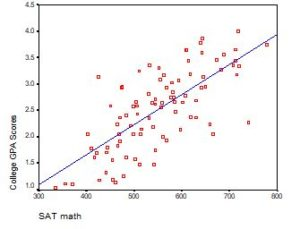# Logistic Regression

### Member Training: Linear Model Assumption Violations: What’s Next?

June 30th, 2023 byWhat do you do if the assumptions of linear models are violated?
(more…)

### Member Training: Interactions in Poisson and Logistic Regression

May 1st, 2023 byInteractions in statistical models are never especially easy to interpret. Throw in non-normal outcome variables and non-linear prediction functions and they become even more difficult to understand. (more…)

### Interpreting the Intercept in a Regression Model

February 21st, 2023 by

Interpreting the Intercept in a regression model isn’t always as straightforward as it looks.

Here’s the definition: the intercept (often labeled the constant) is the expected value of Y when all X=0. But that definition isn’t always helpful. So what does it really mean?

### Regression with One Predictor XIf X sometimes equals 0, the intercept is simply the expected value of Y at that value. In other words, it’s the mean of Y at one value of X. That’s meaningful.

If X never equals 0, then the intercept has no intrinsic meaning. You literally can’t interpret it. That’s actually fine, though. You still need that intercept to give you unbiased estimates of the slope and to calculate accurate predicted values. So while the intercept has a purpose, it’s not meaningful.

Both these scenarios are common in real data. (more…)

### Member Training: Multinomial Logistic Regression

December 30th, 2022 byMultinomial logistic regression is an important type of categorical data analysis. Specifically, it’s used when your response variable is nominal: more than two categories and not ordered.
(more…)

### When Linear Models Don’t Fit Your Data, Now What?

June 20th, 2022 by

When your dependent variable is not continuous, unbounded, and measured onan interval or ratio scale, linear models don’t fit. The data just will not meet the assumptions of linear models. But there’s good news, other models exist for many types of dependent variables.

Today I’m going to go into more detail about 6 common types of dependent variables that are either discrete, bounded, or measured on a nominal or ordinal scale and the tests that work for them instead. Some are all of these.

### Guidelines for writing up three types of odds ratios

December 6th, 2021 by

Odds ratios have a unique part to play in describing the effects of logistic regression models. But that doesn’t mean they’re easy to communicate to an audience who is likely to misinterpret them. So writing up your odds ratios has to be done with care. (more…)